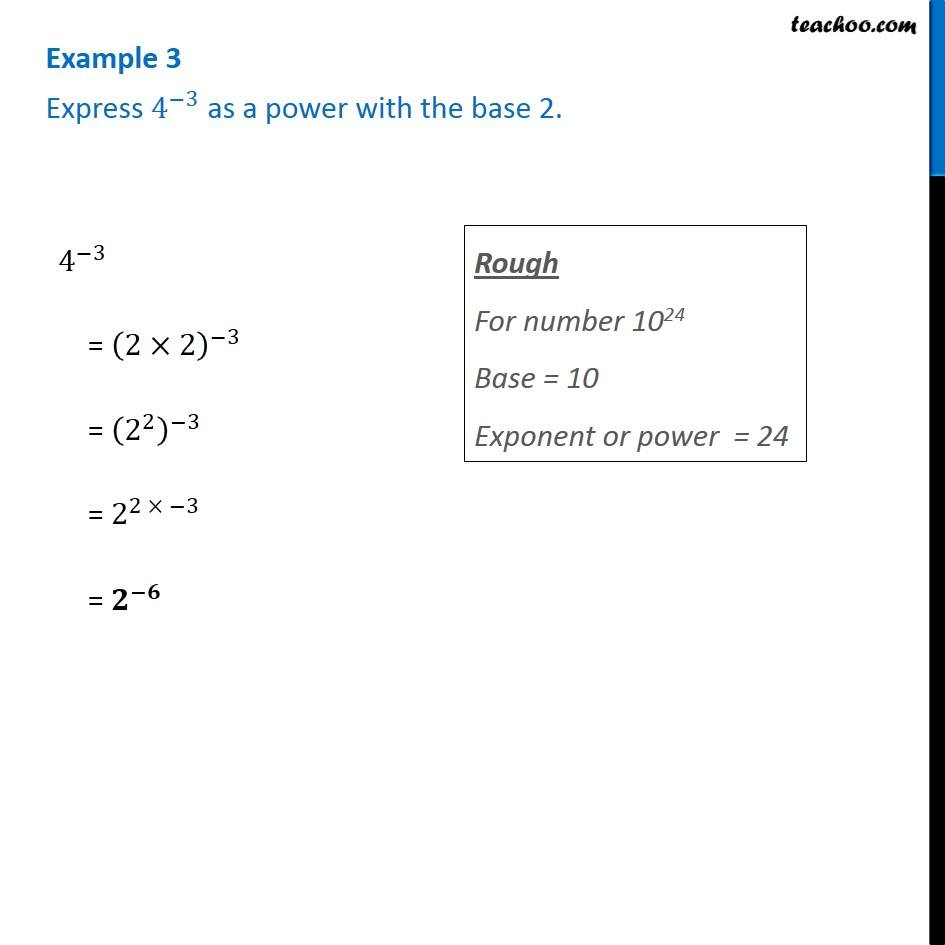Examples

Chapter 10 Class 8 Exponents and Powers
Serial order wiseLearn in your speed, with individual attention - Teachoo Maths 1-on-1 Class

### Transcript

Example 3 Express 4^(−3) as a power with the base 2. 4^(−3) = (2×2)^(−3) = (2^2 )^(−3) = 2^(2 × −3) = 𝟐^(−𝟔) Rough For number 1024 Base = 10 Exponent or power = 24